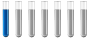Calculating yield based on weightAuthor Message

NewbieJoined: 31 May 2018
Posts: 2Calculating yield based on weight

Hello peeps.

How should I calculate a yield based on weight?

Lets say I have put in 25g of Na2WO4, mixed it up with H3PO4 and crashed out the compound with KCl.

Compounds formula K6[P2W18O64]. Yield = 7g

I want to calculate yield based on the weight of W.

Thanks in advance.Distinguished MemberJoined: 27 Sep 2017
Posts: 287
Location: Berlin, GermanyCalculate: m of your product divided by molar mass of the product gives the moles. The mass of tungsten is then calculated: moles multiplied by the molar mass of tungsten times 18 7g /M(K6[P2W18O64]) = x g /(18* M(W))NewbieJoined: 31 May 2018
Posts: 2Thanks for the quick reply. So I calculated 7g/M(K6[P2W18O64]) = 0.0015 mol 18*M(W) = 3311.11 g/mol Then x = 0.0015 mol * 3311.11 g/mol = 4.96g As I understand x is W weight in my product mixture. How do I calculate the yield percentage now? Is it m(W product mixture) / m(W in 25g of Na2WO4) * 100% ?Distinguished MemberJoined: 27 Sep 2017
Posts: 287
Location: Berlin, GermanyNo you have to calculate the m of W in Na2WO4 first. Then you divide this value by the m of W of your product.Display posts from previous: All Posts1 Day7 Days2 Weeks1 Month3 Months6 Months1 Year Oldest FirstNewest FirstPage 1 of 1